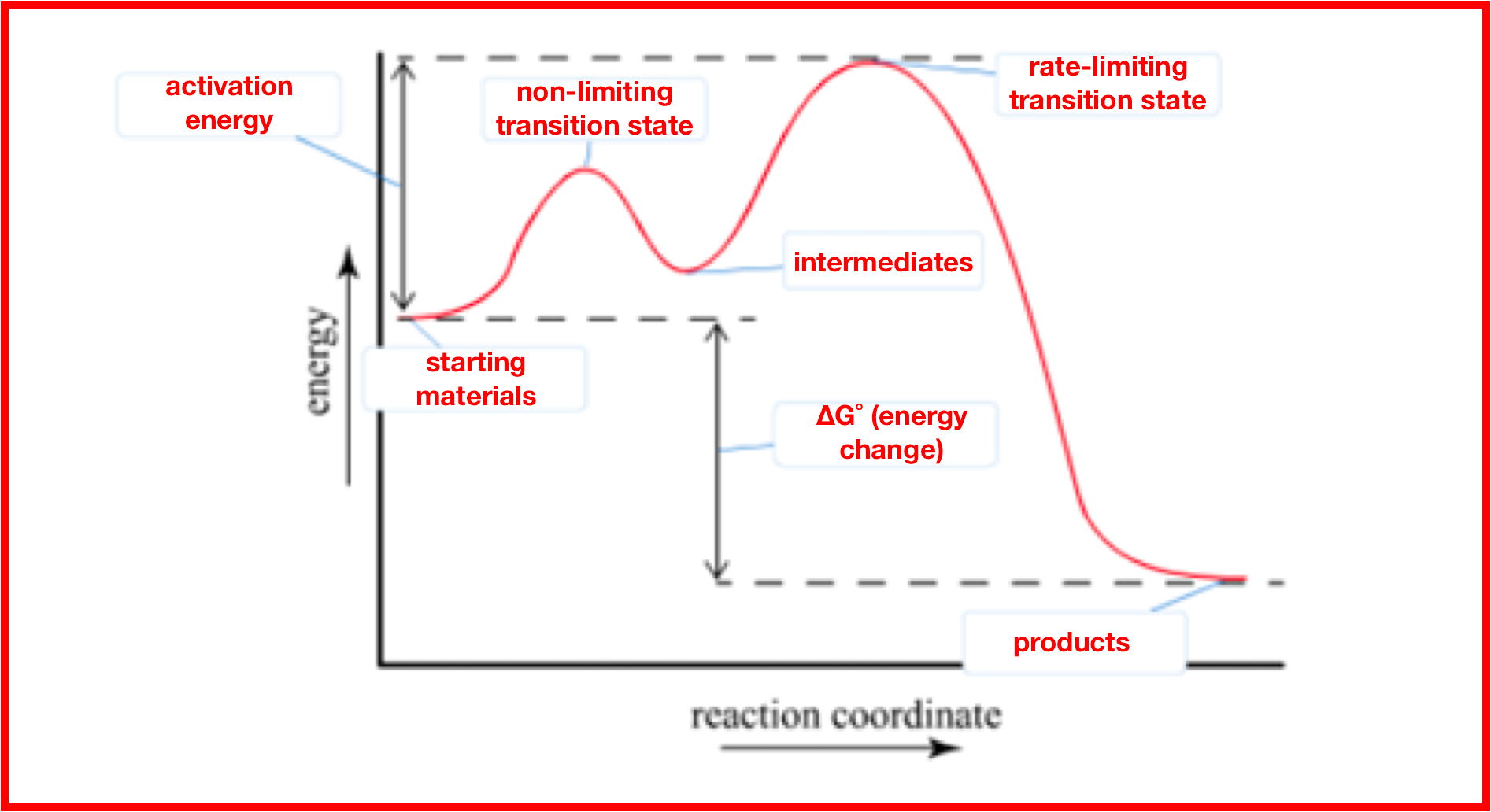# Problem: A chemical reaction is endothermic and has an activation energy that is twice the value of the enthalpy of the reaction. Draw a diagram depicting the energy of the reaction as it progresses. Label the position of the reactants and products and indicate the activation energy and enthalpy of reaction.

###### FREE Expert Solution

Recall that an energy diagram is usually read from left to right

The components of a two-step energy diagram are:

• Reactants: are placed on the left/beginning of the energy diagram

• Products: are placed on the right/end of the energy diagram

• Non-limiting transition state: is the transition state with the lowest energy in the energy diagram

• Rate-limiting transition state: is the transition state with the highest energy in the energy diagram

• Intermediates: are formed in between steps in the energy diagram

• Energy change (ΔH or ΔG˚): is the difference in energy between products and reactants

• Activation energy (Ea): is the difference in energy between reactants and transition state

Below is an example of a labelled diagram:For the given conditions, below is the energy diagram being described:

99% (127 ratings)###### Problem Details

A chemical reaction is endothermic and has an activation energy that is twice the value of the enthalpy of the reaction. Draw a diagram depicting the energy of the reaction as it progresses. Label the position of the reactants and products and indicate the activation energy and enthalpy of reaction.